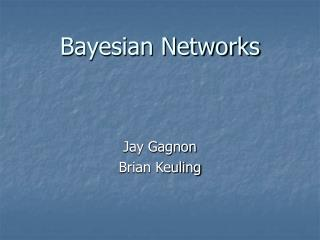DownloadDownload PresentationBayesian Networks

# Bayesian Networks

Télécharger la présentation## Bayesian Networks

- - - - - - - - - - - - - - - - - - - - - - - - - - - E N D - - - - - - - - - - - - - - - - - - - - - - - - - - -
##### Presentation Transcript

1. Bayesian Networks Jay Gagnon Brian Keuling

2. Bayesian Networks Directed Acyclic Graph (DAG) Nodes = variables Arcs = dependence relationship Conditional probability associated with nodes Nodes with parents are called conditional Nodes without parents are called unconditional

3. Graph Example

4. Bayes’ Theorem Reverend Thomas Bayes (1702 - 1761) Uses “inverse probability” Probabilities of previous events affect the probability of a future event Bayes’ Rule: P(A|B) = [P(B|A) x P(A)] / P(B) The probability of A given B equals the probability of B given A times the probability of A, divided by the probability of B. posterior = likelihood x prior / normalizing constant

5. Graph Example Suppose that P(rain today) = 0.20 and P(rain tomorrow given that it rains today) = 0.70 E1 = rain today, E2 = rain tomorrow P(E1,E2) = P(E1) * P(E2|E1)

6. Joint Distributions The joint distribution for the set of variables U = {C,S1, S2, S3} from Figure 1 is specified as p(U) = p(C) p(S1|C) p(S2|C) p(S3|C).

7. Applications Google search engine Spam filter to remove unwanted pages from search results AI (decision making) Spam filters Breaks emails down into words and tokens Learns which tokens indicate spam and which indicate valid emails Harder to cheat, multi-lingual, incredibly effective when properly “trained” Microsoft Notification Platform E-mail and cell phone notifications Example on next Slide

8. Applications (continued) Program observes user activity Email response time, keyboard activity, meetings Program creates Bayesian Network When emails arrive, program can use BN to determine appropriate action Email from “The Boss” vs from a middle manager

9. Bibliography http://www.csse.monash.edu.au/bai/ http://www.ia.uned.es/~fjdiez/bayes/ http://www.niedermayer.ca/papers/bayesian/bayes.html http://en.wikipedia.org/wiki/Bayesian_networks http://site.ebrary.com.online.library.marist.edu/lib/marist/Doc?id=10047051 http://www.cs.ubc.ca/~murphyk/Bayes/Charniak_91.pdf http://www.gfi.com/whitepapers/why-bayesian-filtering.pdf http://www.cs.ubc.ca/spider/poole/papers/canai94.pdf http://www.cs.ualberta.ca/~greiner/bn.html http://www.cs.ubc.ca/~murphyk/Software/BNT/bnsoft.html http://www.cs.huji.ac.il/~nir/Nips01-Tutorial/ http://ite.gmu.edu/~klaskey/papers/gecco99.pdf http://news.com.com/Old-school+theory+is+a+new+force/2009-1001_3-984695.html